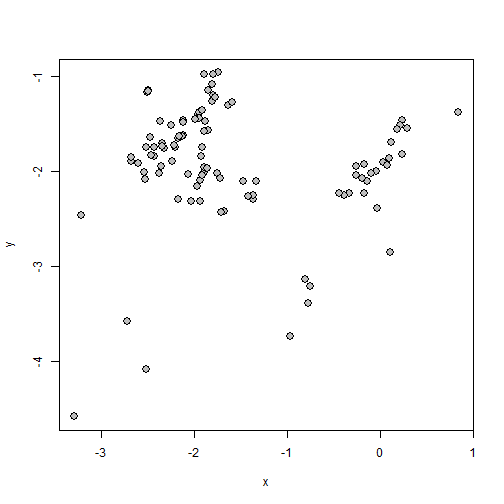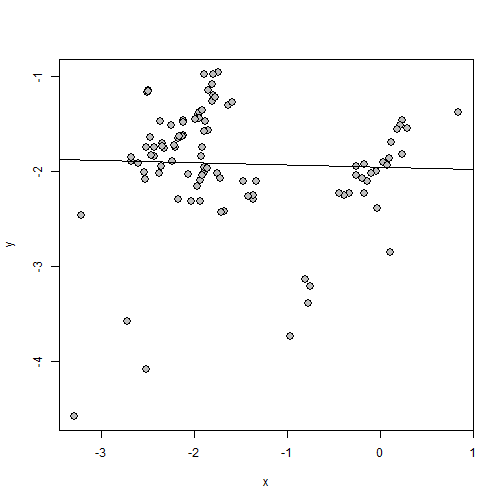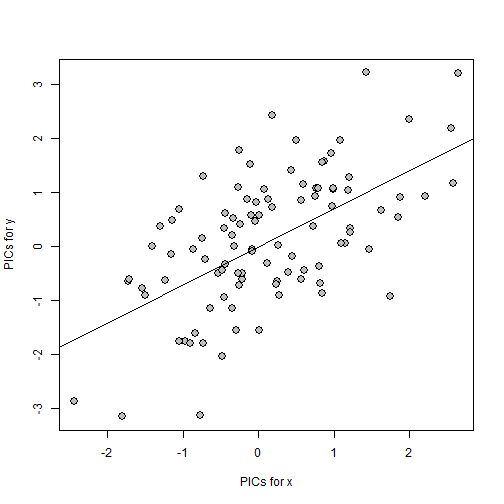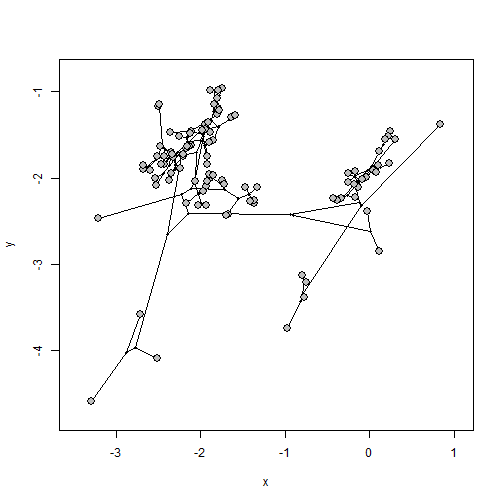Solution to Challenge Problem 1: Phylogenetically Independent Contrasts

This first challenge problem asked you to download the two files (pic-exercise-data.csv and pic-exercise-tree.tre) then fit an OLS (non-phylogenetic) regression model for `y~x` and a phylogenetic regression model using contrasts, as well as to explain what you found.

Here is my solution. First, load packages:

``````## load phytools
library(phytools)
``````
``````## Loading required package: ape
``````
``````## Loading required package: maps
``````

Read the data and tree from file:

``````## load data
X<-read.csv("pic-exercise-data.csv",row.names=1)
## load tree
tree<-read.tree("pic-exercise-tree.tre")
``````

This is not strictly necessary, but it will make things easier if we pull out the columns of `X` as separate vectors:

``````x<-X[,1]
names(x)<-rownames(X)
## or
y<-setNames(X[,2],rownames(X))
``````

Now, let's plot `x` & `y`:

``````plot(x,y,pch=21,bg="grey",cex=1.5)
``````Looks like there is no relationship, right?

``````fit<-lm(y~x)
plot(x,y,pch=21,bg="grey",cex=1.5)
abline(fit)
````````````fit
``````
``````##
## Call:
## lm(formula = y ~ x)
##
## Coefficients:
## (Intercept)            x
##    -1.95546     -0.02282
``````
``````summary(fit)
``````
``````##
## Call:
## lm(formula = y ~ x)
##
## Residuals:
##      Min       1Q   Median       3Q      Max
## -2.70042 -0.18339  0.04348  0.41484  0.95401
##
## Coefficients:
##             Estimate Std. Error t value Pr(>|t|)
## (Intercept) -1.95546    0.12017 -16.272   <2e-16 ***
## x           -0.02282    0.06582  -0.347     0.73
## ---
## Signif. codes:  0 '***' 0.001 '**' 0.01 '*' 0.05 '.' 0.1 ' ' 1
##
## Residual standard error: 0.6289 on 98 degrees of freedom
## Multiple R-squared:  0.001225,   Adjusted R-squared:  -0.008967
## F-statistic: 0.1202 on 1 and 98 DF,  p-value: 0.7296
``````
``````anova(fit)
``````
``````## Analysis of Variance Table
##
## Response: y
##           Df Sum Sq Mean Sq F value Pr(>F)
## x          1  0.048 0.04753  0.1202 0.7296
## Residuals 98 38.763 0.39554
``````

However, we cannot forget that our data are related by a phylogenetic tree. Let's account for that covariance among the data in `x` & `y` using the contrasts method:

``````## compute contrasts
icx<-pic(x,tree)
icy<-pic(y,tree)
## plot contrasts
plot(icx,icy,pch=21,bg="grey",cex=1.5,
xlab="PICs for x",ylab="PICs for y")
## fit model without intercept
fit<-lm(icy~icx-1)
abline(fit)
````````````summary(fit)
``````
``````##
## Call:
## lm(formula = icy ~ icx - 1)
##
## Residuals:
##     Min      1Q  Median      3Q     Max
## -2.5957 -0.7257  0.2020  0.7100  2.3120
##
## Coefficients:
##     Estimate Std. Error t value Pr(>|t|)
## icx  0.70387    0.09648   7.296 7.81e-11 ***
## ---
## Signif. codes:  0 '***' 0.001 '**' 0.01 '*' 0.05 '.' 0.1 ' ' 1
##
## Residual standard error: 0.9944 on 98 degrees of freedom
## Multiple R-squared:  0.352,  Adjusted R-squared:  0.3454
## F-statistic: 53.23 on 1 and 98 DF,  p-value: 7.808e-11
``````

How can we account for this? Well, if we project our tree into the phenotype space, we will see that within each clade there is a relationship, but our ability to measure this relationship breaks down due to differences between clades:

``````phylomorphospace(tree,cbind(x,y),label="off",node.size=c(0.5,0.7))
points(x,y,pch=21,bg="grey",cex=1.5)
``````Written by Liam J. Revell. Last updated 27 June 2018.